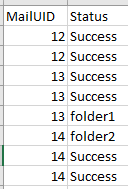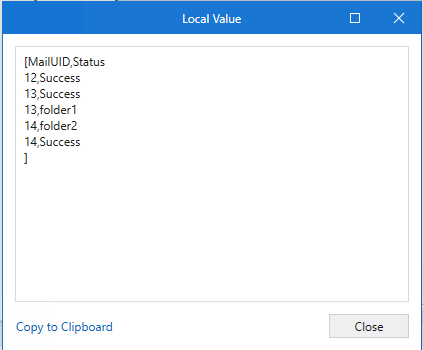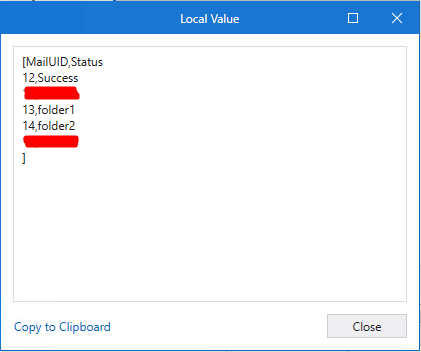# How to use group by and if activities together with linq?

Hi,

I have a data as follows:I need to group this data according to the status.I need to group this data according to the status. And then if two statuses belonging to the same MailUID are found; I want it to write the value that is “folderx”, not the value that is Success.

The linq expression I used is below. How do I do that?

``````(
From x In dt_DataTable
Group x By a = x("MailUID").ToString.Trim, b = x("Status").ToString.Trim Into Group
).CopyToDataTable
``````

The format I want:Thanks.

Hi,

Can you try the following expression?

``````dt = dt.AsEnumerable.GroupBy(Function(r) r("MailUID").ToString()).SelectMany(Function(g) g.Where(Function(r,i) r("Status").ToString.Trim<>"Success" OrElse (i=0 AndAlso g.All(Function(r2) r2("Status").ToString.Trim="Success")))).CopyToDataTable()
``````

Sample220221113-2L.zip (2.9 KB)

Regards,

1 Like

This topic was automatically closed 3 days after the last reply. New replies are no longer allowed.